# Number System conversions Number Systems There are four

• Slides: 46Number System conversionsNumber Systems � There are four systems of arithmetic which are often used in digital electronics. ◦ ◦ Decimal Number System Binary Number System Octal Number System Hexa Decimal System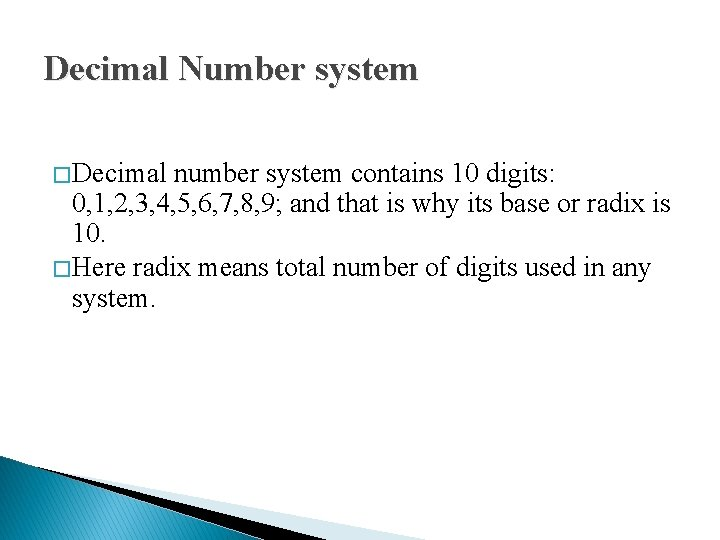Decimal Number system � Decimal number system contains 10 digits: 0, 1, 2, 3, 4, 5, 6, 7, 8, 9; and that is why its base or radix is 10. � Here radix means total number of digits used in any system.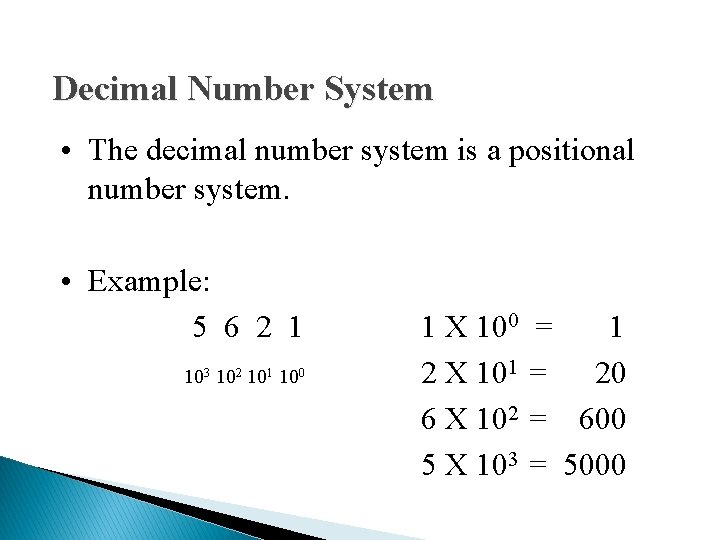Decimal Number System • The decimal number system is a positional number system. • Example: 5 6 2 1 103 102 101 100 1 X 100 = 1 2 X 101 = 20 6 X 102 = 600 5 X 103 = 5000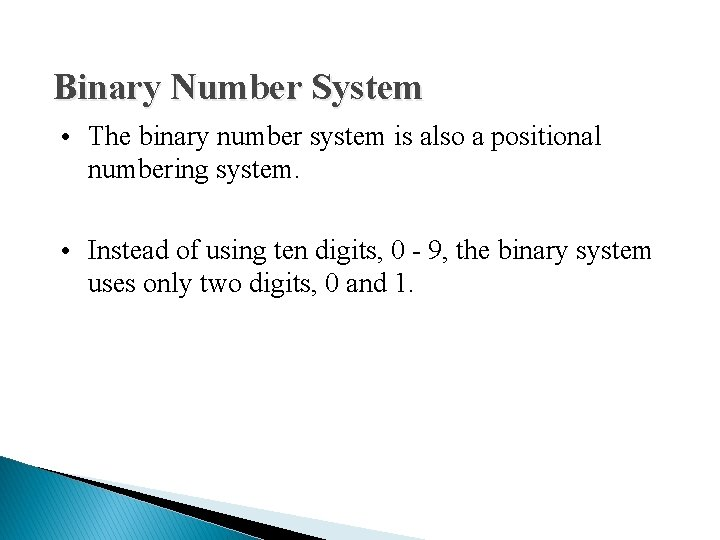Binary Number System • The binary number system is also a positional numbering system. • Instead of using ten digits, 0 - 9, the binary system uses only two digits, 0 and 1.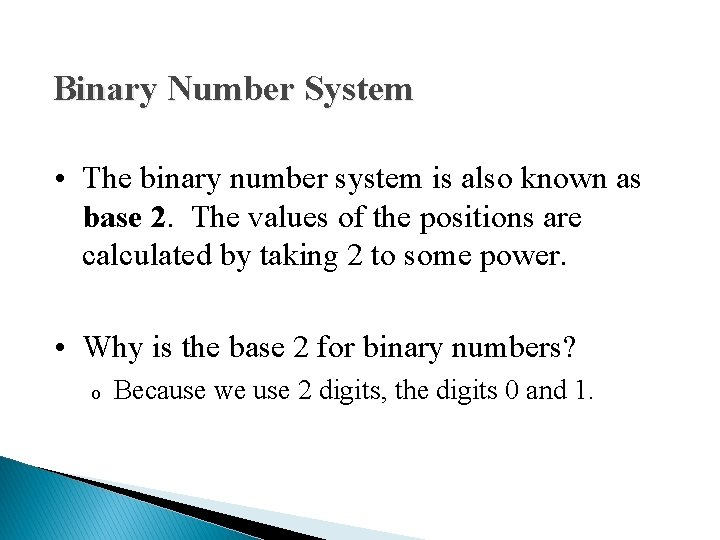Binary Number System • The binary number system is also known as base 2. The values of the positions are calculated by taking 2 to some power. • Why is the base 2 for binary numbers? o Because we use 2 digits, the digits 0 and 1.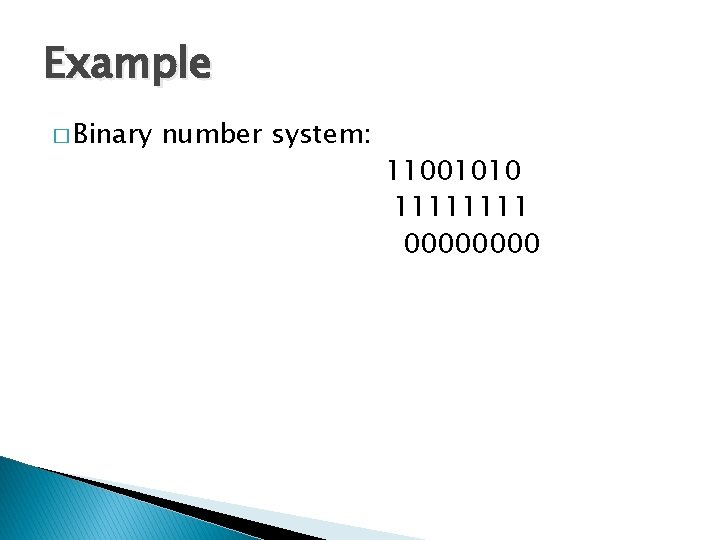Example � Binary number system: 11001010 1111 0000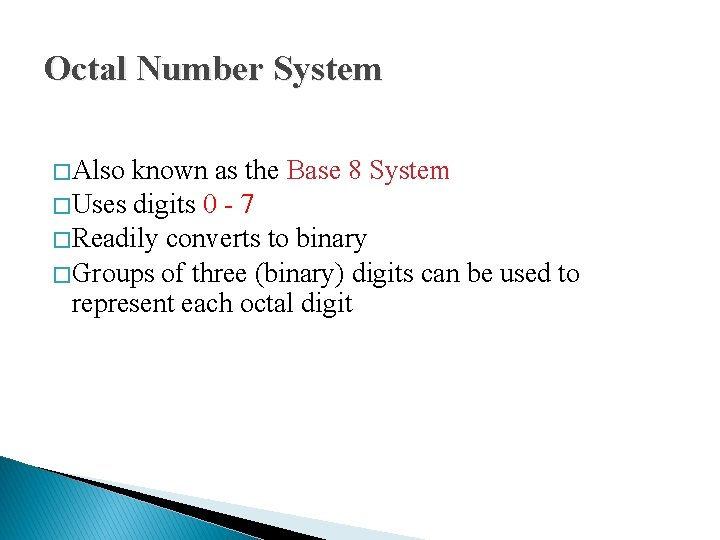Octal Number System � Also known as the Base 8 System � Uses digits 0 - 7 � Readily converts to binary � Groups of three (binary) digits can be used to represent each octal digit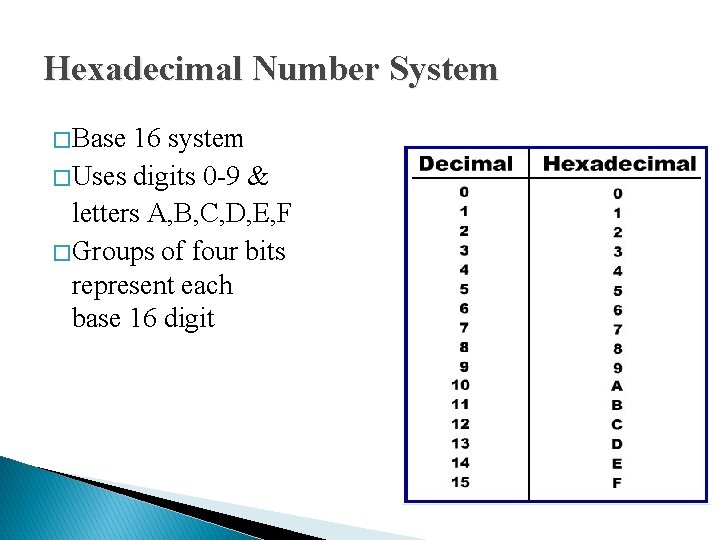Hexadecimal Number System � Base 16 system � Uses digits 0 -9 & letters A, B, C, D, E, F � Groups of four bits represent each base 16 digit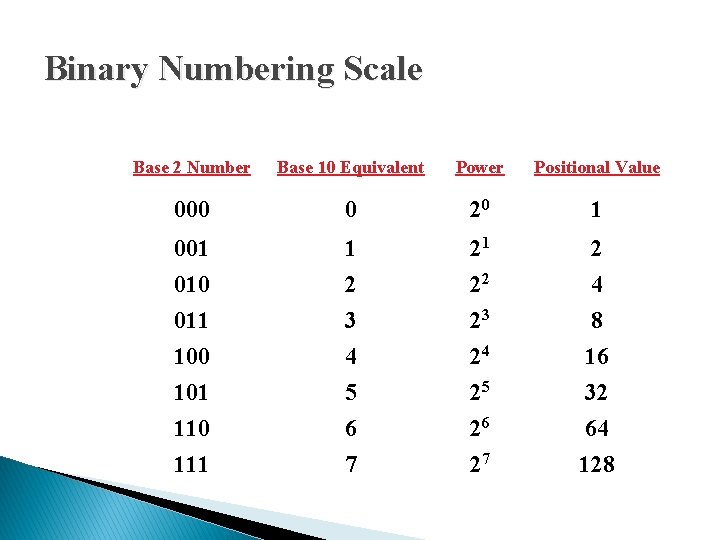Binary Numbering Scale Base 2 Number Base 10 Equivalent Power Positional Value 000 0 20 1 001 010 011 100 101 110 1 2 3 4 5 6 21 22 23 24 25 26 2 4 8 16 32 64 111 7 27 128Significant Digits Binary: 11101101 Most significant digit Least significant Hexadecimal: 1 D 63 A 7 A Most significant digit Least significant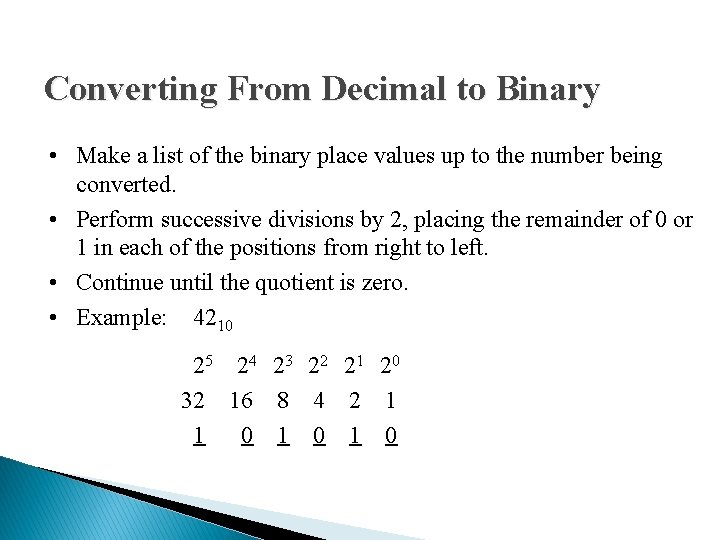Converting From Decimal to Binary • Make a list of the binary place values up to the number being converted. • Perform successive divisions by 2, placing the remainder of 0 or 1 in each of the positions from right to left. • Continue until the quotient is zero. • Example: 4210 25 24 23 22 21 20 32 16 8 4 2 1 1 0 1 0DECIMAL TO BINARY CONVERTION � There are two methods to convert it: - i. Revese of Binary-To-Digital Method ü Decimal number write as the sum of square ü 0 & 1 is write on the byte Example 1: Convert 4510 to the binary value Solve = = 32 + 8 + 4 + 1 0 0 = 1 = 0 1 1 0 12ii. Repeat division method ü The numbers is divide by 2. ü Balance for the question is written until the last answer. Example : convert 2510 to binary Solve = 2510 = ? 2 25 = 2 12 balance 1 = 12 6 balance 0 2 = 62 3 balance 0 = 32 1 balance 1 = 12 0 balance 1. . . Answer = 110012 LSB MSB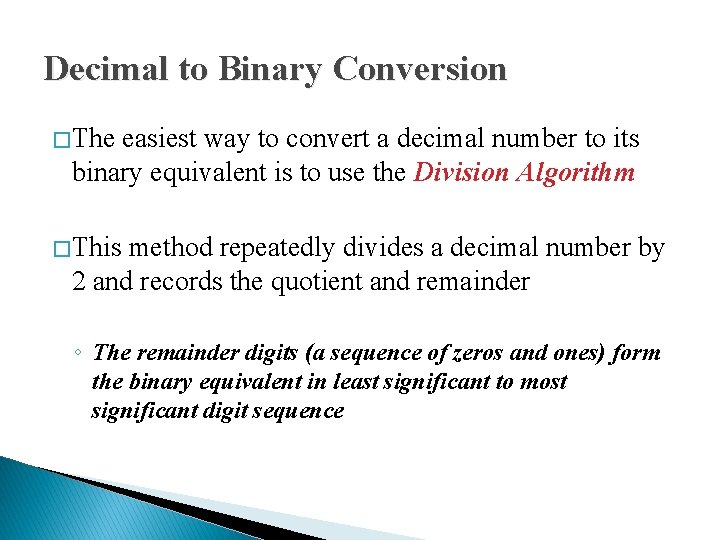Decimal to Binary Conversion � The easiest way to convert a decimal number to its binary equivalent is to use the Division Algorithm � This method repeatedly divides a decimal number by 2 and records the quotient and remainder ◦ The remainder digits (a sequence of zeros and ones) form the binary equivalent in least significant to most significant digit sequence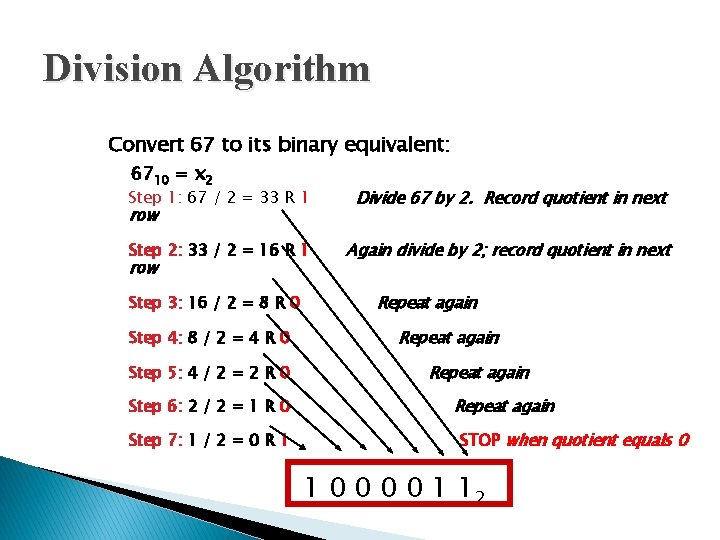Division Algorithm Convert 67 to its binary equivalent: 6710 = x 2 Step 1: 67 / 2 = 33 R 1 Divide 67 by 2. Record quotient in next Step 2: 33 / 2 = 16 R 1 Again divide by 2; record quotient in next row Step 3: 16 / 2 = 8 R 0 Step 4: 8 / 2 = 4 R 0 Step 5: 4 / 2 = 2 R 0 Step 6: 2 / 2 = 1 R 0 Step 7: 1 / 2 = 0 R 1 Repeat again STOP when quotient equals 0 1 0 0 1 12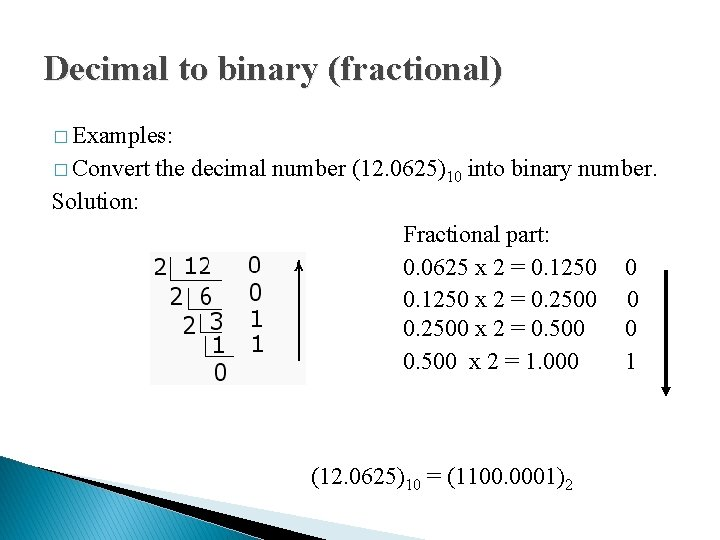Decimal to binary (fractional) � Examples: � Convert the decimal number (12. 0625)10 into binary number. Solution: Fractional part: 0. 0625 x 2 = 0. 1250 0 0. 1250 x 2 = 0. 2500 0 0. 2500 x 2 = 0. 500 0 0. 500 x 2 = 1. 000 1 (12. 0625)10 = (1100. 0001)2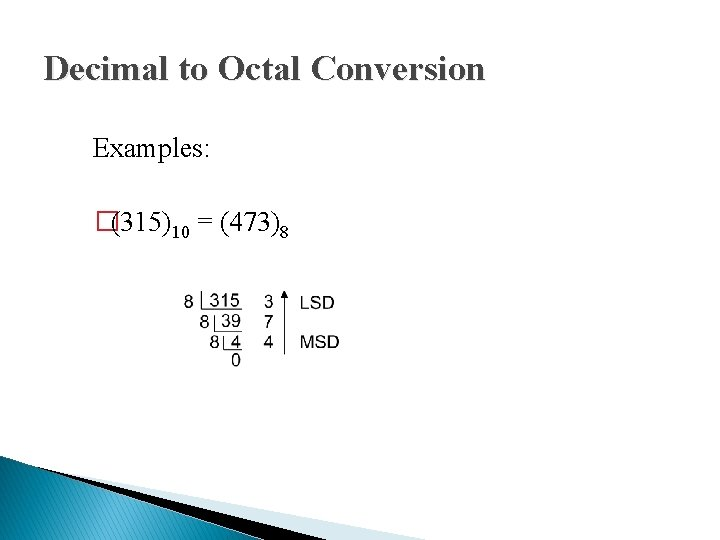Decimal to Octal Conversion Examples: �(315)10 = (473)8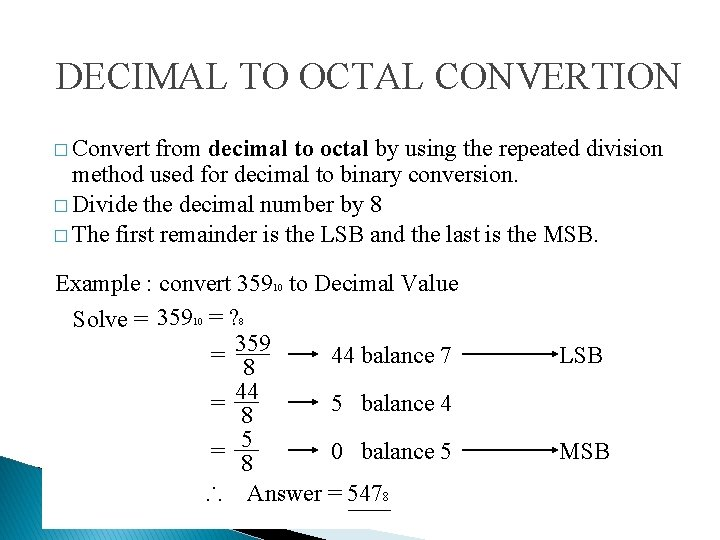DECIMAL TO OCTAL CONVERTION � Convert from decimal to octal by using the repeated division method used for decimal to binary conversion. � Divide the decimal number by 8 � The first remainder is the LSB and the last is the MSB. Example : convert 35910 to Decimal Value Solve = 35910 = ? 8 = 359 44 balance 7 8 = 44 5 balance 4 8 = 58 0 balance 5. . . Answer = 5478 LSB MSB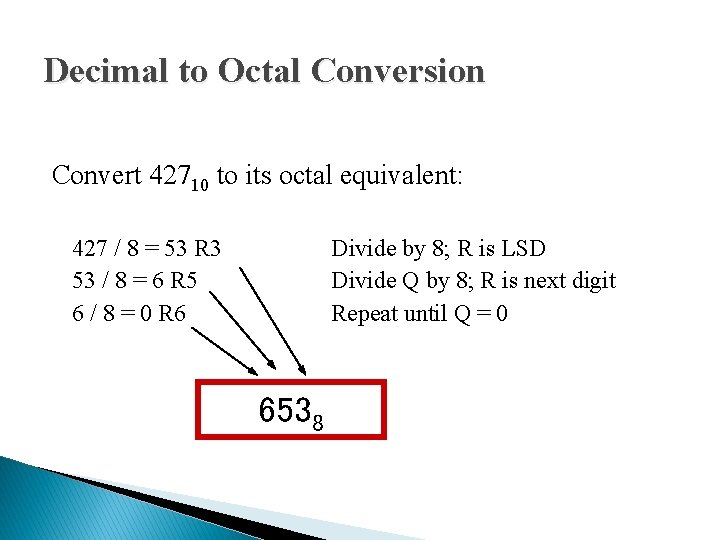Decimal to Octal Conversion Convert 42710 to its octal equivalent: 427 / 8 = 53 R 3 53 / 8 = 6 R 5 6 / 8 = 0 R 6 Divide by 8; R is LSD Divide Q by 8; R is next digit Repeat until Q = 0 6538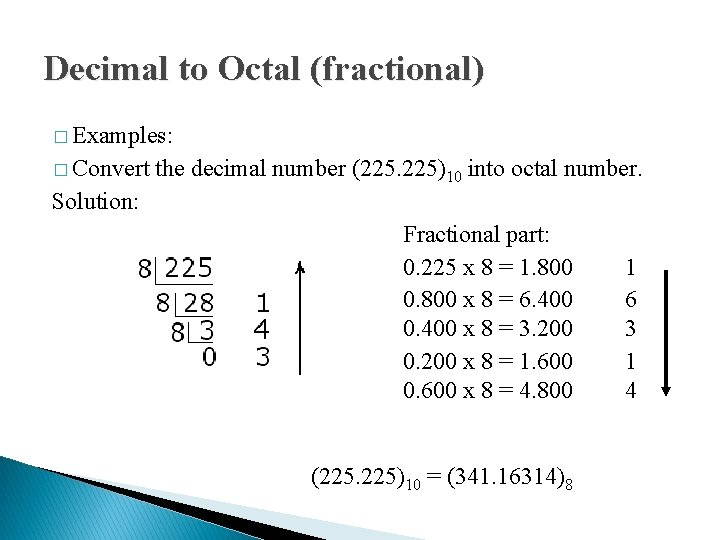Decimal to Octal (fractional) � Examples: � Convert the decimal number (225. 225)10 into octal number. Solution: Fractional part: 0. 225 x 8 = 1. 800 0. 800 x 8 = 6. 400 0. 400 x 8 = 3. 200 0. 200 x 8 = 1. 600 0. 600 x 8 = 4. 800 (225. 225)10 = (341. 16314)8 1 6 3 1 4Decimal to Hexadecimal Conversion ◦ Examples �(315)10 = (13 B)16Decimal to Hexadecimal Conversion Convert 83010 to its hexadecimal equivalent: 830 / 16 = 51 R 14 51 / 16 = 3 R 3 3 / 16 = 0 R 3 = E in Hex 33 E 16Decimal to Hexadecimal (fractional) � Examples: � Convert the decimal number (225. 225)10 into hexadecimal number. Solution: Fractional part: 0. 225 x 16 = 3. 600 0. 600 x 16 = 9. 600 (225. 225)10 = (E 1. 39)16 3 9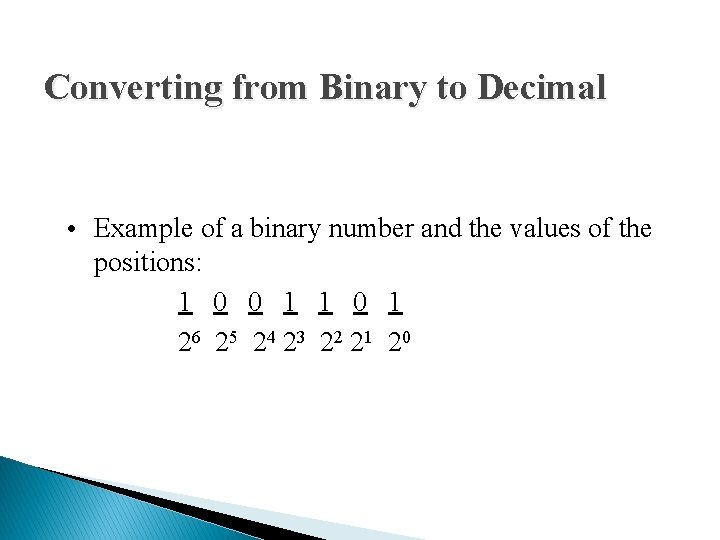Converting from Binary to Decimal • Example of a binary number and the values of the positions: 1 0 0 1 1 0 1 26 25 24 23 22 21 20Converting from Binary to Decimal 1 0 0 1 1 0 1 26 25 24 23 22 21 20 20 = 1 24 = 16 21 = 2 25 = 32 26 = 64 22 = 4 23 = 8 1 X 20 = 1 0 X 21 = 0 1 X 22 = 4 1 X 23 = 8 0 X 24 = 0 0 X 25 = 0 1 X 26 = 64 7710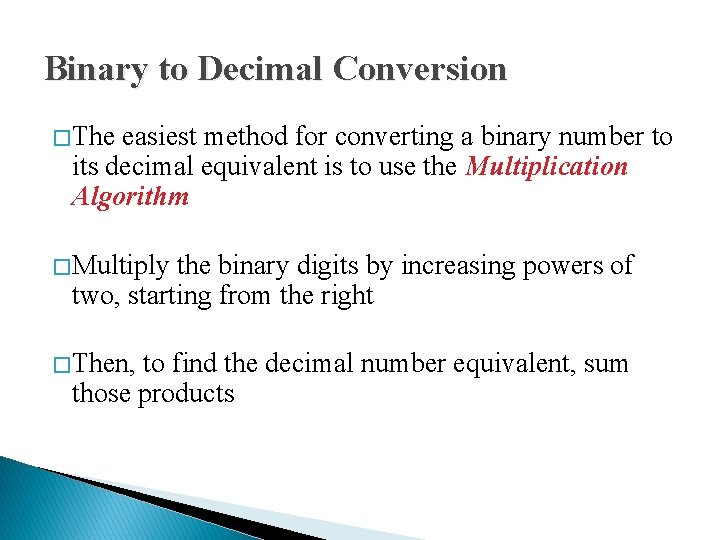Binary to Decimal Conversion � The easiest method for converting a binary number to its decimal equivalent is to use the Multiplication Algorithm � Multiply the binary digits by increasing powers of two, starting from the right � Then, to find the decimal number equivalent, sum those productsMultiplication Algorithm Convert (10101101)2 to its decimal equivalent: Binary Positional Values Products 1 0 1 x x x x 27 26 25 24 23 22 21 20 128 + 32 + 8 + 4 + 1 17310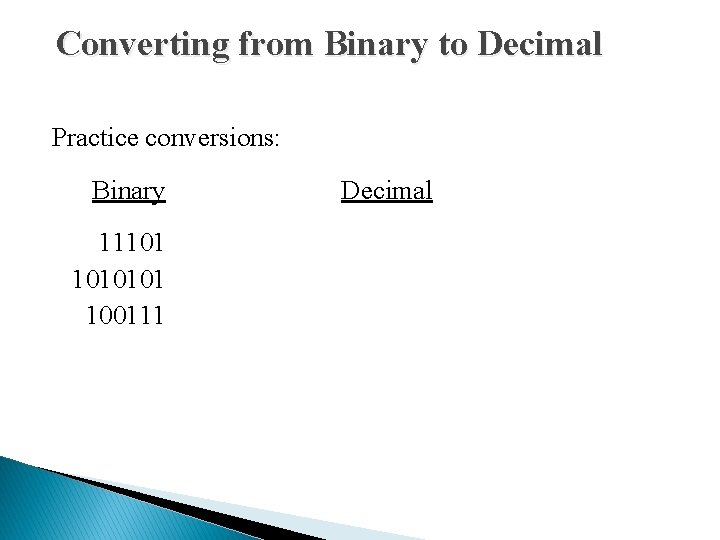Converting from Binary to Decimal Practice conversions: Binary Decimal 11101 1010101 100111Converting From Decimal to Binary Practice conversions: Decimal Binary 59 82 175BINARY TO OCTAL CONVERSION � Can be converted by grouping the binary bit in group of three starting from LSB � Octal is a base-8 system and equal to two the power of three, so a digit in Octal is equal to three digit in binary system.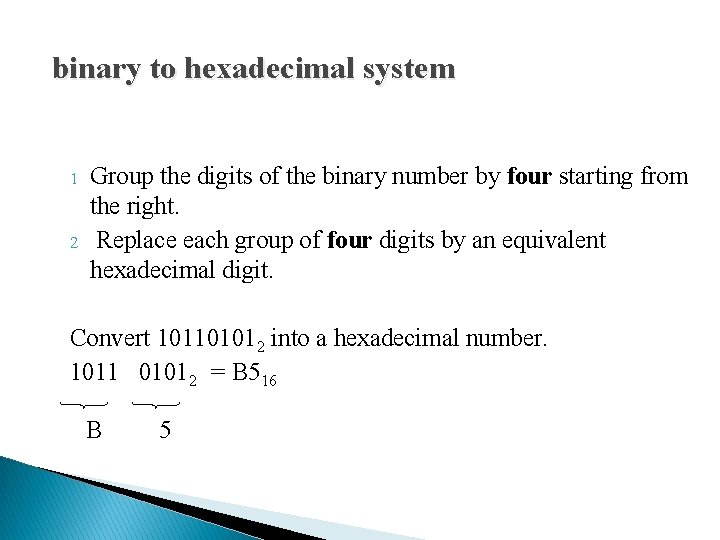binary to hexadecimal system 1 2 Group the digits of the binary number by four starting from the right. Replace each group of four digits by an equivalent hexadecimal digit. Convert 101101012 into a hexadecimal number. 1011 01012 = B 516 B 5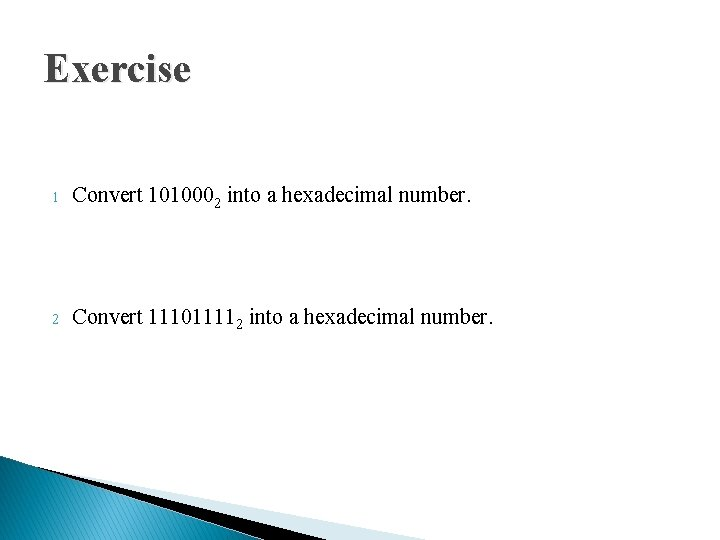Exercise 1 Convert 1010002 into a hexadecimal number. 2 Convert 111011112 into a hexadecimal number.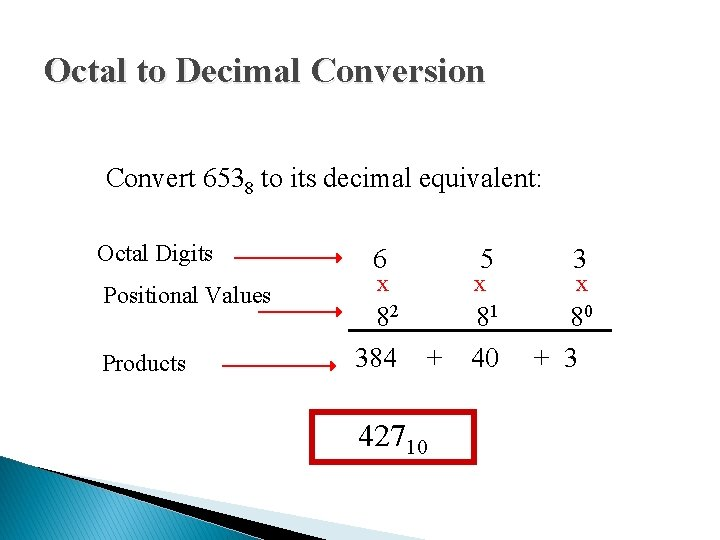Octal to Decimal Conversion Convert 6538 to its decimal equivalent: Octal Digits Positional Values Products 6 x 5 3 x x 82 81 80 384 + 40 + 3 42710OCTAL TO BINARY CONVERTION � Convert from octal to binary by converting each octal digit to a three bit binary equivalent Octal digit 0 1 2 3 4 5 6 7 Binary Equivalent 000 000 • Convert from binary to octal by grouping bits in threes starting with the LSB. • Each group is then converted to the octal equivalent • Leading zeros can be added to the left of the MSB to fill out the last group.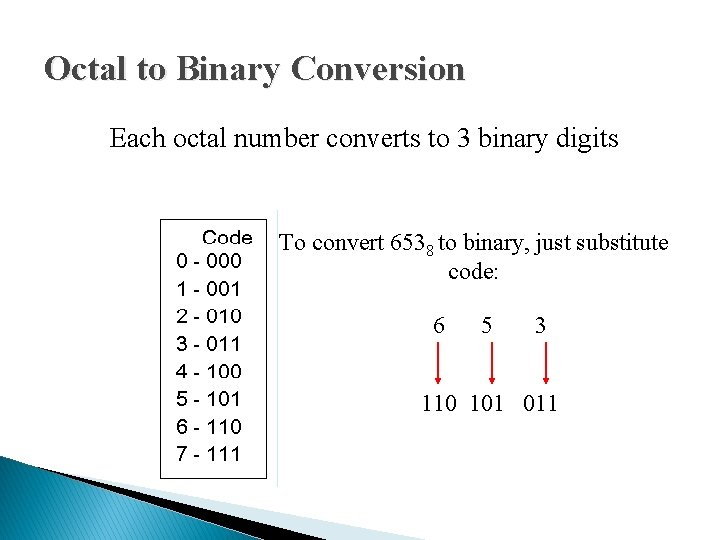Octal to Binary Conversion Each octal number converts to 3 binary digits To convert 6538 to binary, just substitute code: 6 5 3 110 101 011Hexadecimal to Decimal Conversion Convert 3 B 4 F 16 to its decimal equivalent: Hex Digits 3 B 4 F Positional Values Products x x 163 162 161 160 12288 +2816 + 64 +15 15, 18310HEXADECIMAL TO binary � To convert a hexadecimal to binary number, convert each hexadecimal digit to its 4 bit equivalent using the hexa number. • Example: (23. AB)16 = ()2 Solution: (23. AB)16 = 2 3 . A B 0010 0011 1010 1011 (23. AB)16 = (00100011. 10101011)2HEXADECIMAL TO OCTAL CONVERTION � There is two ways to convert it: - i. i. Hexadecimal – Decimal – Octal Hexadecimal – Binary – Octal Hexadecimal – Decimal – Octal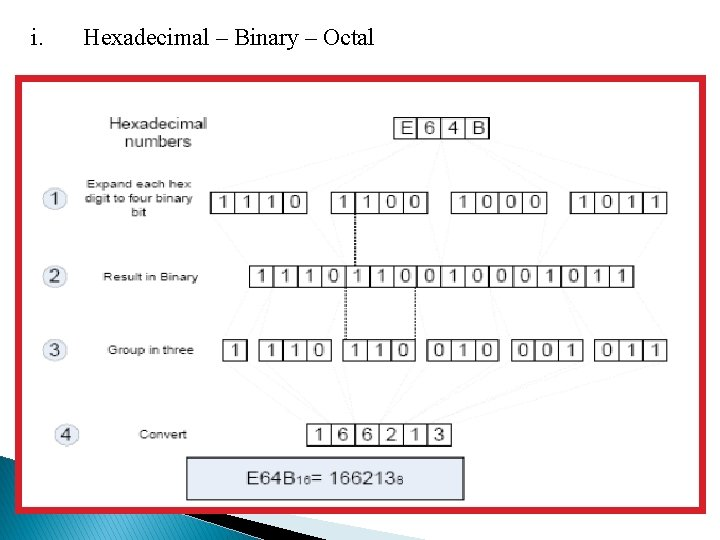i. Hexadecimal – Binary – Octal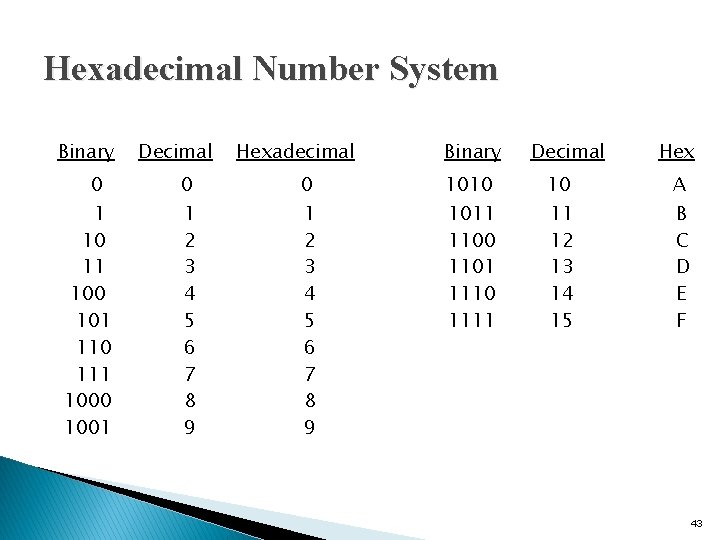Hexadecimal Number System Binary 0 1 10 11 100 101 110 111 1000 1001 Decimal Hexadecimal Binary Decimal Hex 0 0 1010 10 A 1 2 3 4 5 6 7 8 9 1011 1100 1101 1110 1111 11 12 13 14 15 B C D E F 43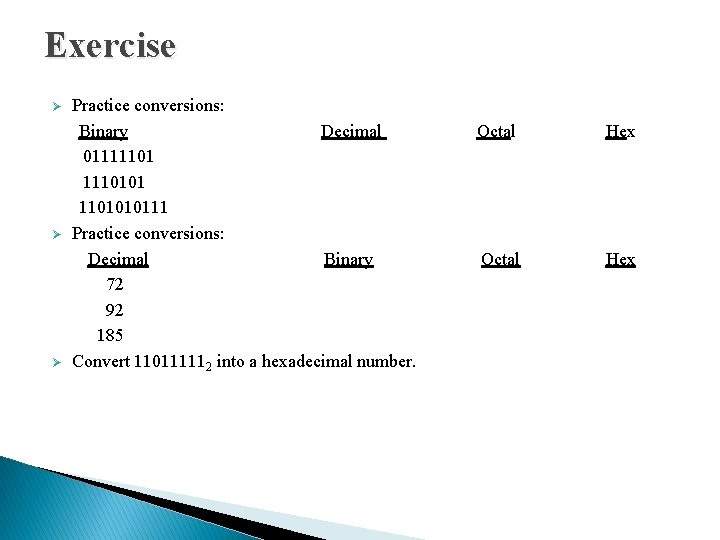Exercise Practice conversions: Binary Decimal Octal Hex 01111101 1110101 1101010111 Ø Practice conversions: Decimal Binary Octal Hex 72 92 185 Ø Convert 110111112 into a hexadecimal number. Ø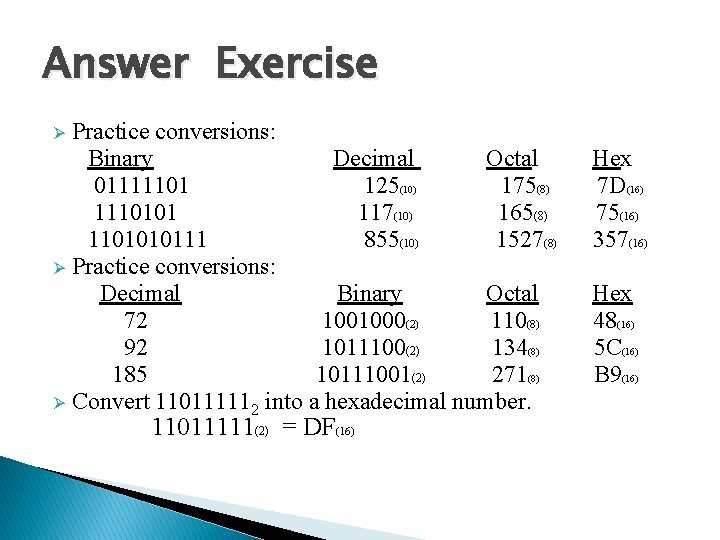Answer Exercise Practice conversions: Binary Decimal Octal Hex 01111101 125(10) 175(8) 7 D(16) 1110101 117(10) 165(8) 75(16) 1101010111 855(10) 1527(8) 357(16) Ø Practice conversions: Decimal Binary Octal Hex 72 1001000(2) 110(8) 48(16) 92 1011100(2) 134(8) 5 C(16) 185 10111001(2) 271(8) B 9(16) Ø Convert 110111112 into a hexadecimal number. Ø 11011111(2) = DF(16)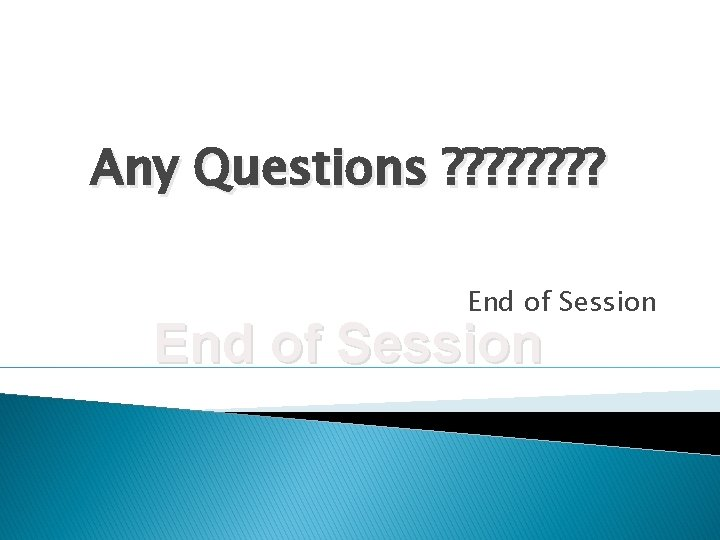Any Questions ? ? ? ? End of Session